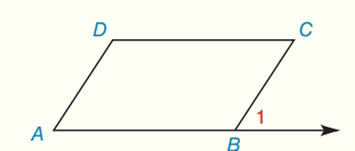Chapter 2.CR, Problem 36CR### Elementary Geometry for College St...

6th Edition
Daniel C. Alexander + 1 other
ISBN: 9781285195698

#### Solutions

Chapter
Section### Elementary Geometry for College St...

6th Edition
Daniel C. Alexander + 1 other
ISBN: 9781285195698
Textbook Problem
1 views

# Given: ∠ A ≅ ∠ C D C ¯ ∥ A B → Prove: D A ¯ ∥ C B ¯To determine

To prove:

DA¯ is parallel to CB¯.

Explanation

Given:

The given statement is,

AC and DC¯AB

Figure (1)

Properties used:

If two parallel lines are cut by a transversal, then the pairs of the alternate interior angles are congruent.

If two lines are cut by a transversal and the pair of corresponding interior angles are congruent, then the lines are parallel.

Approach:

The given statement is,

AC and DC¯AB

C and 1 are alternate interior angles.

So,

C1

Use Transitive property of congruence.

A1

A and 1 are corresponding congruent angles.

So,

DA¯CB¯

The proof for the given statement is shown in the following table,

 Proof Statements Reasons 1

### Still sussing out bartleby?

Check out a sample textbook solution.

See a sample solution

#### The Solution to Your Study Problems

Bartleby provides explanations to thousands of textbook problems written by our experts, many with advanced degrees!

Get Started

#### Find more solutions based on key concepts